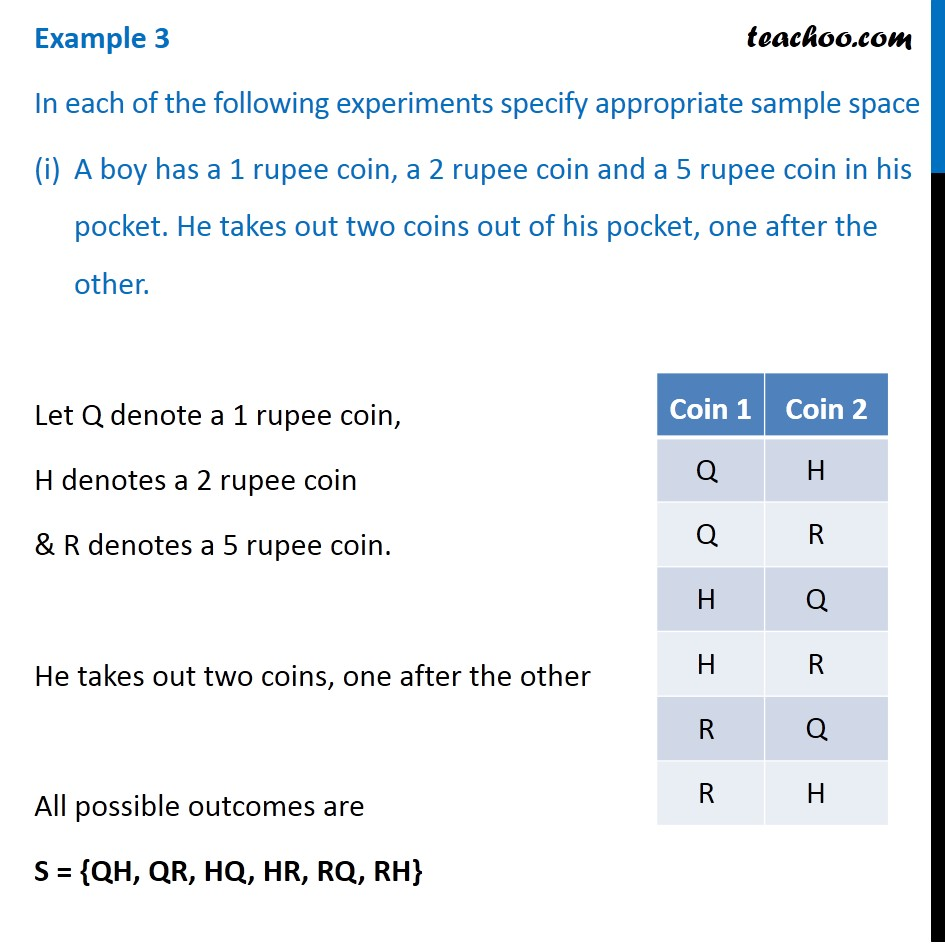Subscribe to our Youtube Channel - https://you.tube/teachoo

1. Chapter 16 Class 11 Probability
2. Concept wise
3. Sample Space

Transcript

Example 3 In each of the following experiments specify appropriate sample space A boy has a 1 rupee coin, a 2 rupee coin and a 5 rupee coin in his pocket. He takes out two coins out of his pocket, one after the other. Let Q denote a 1 rupee coin, H denotes a 2 rupee coin & R denotes a 5 rupee coin. He takes out two coins, one after the other All possible outcomes are S = {QH, QR, HQ, HR, RQ, RH} Example 3 In each of following experiments specify appropriate sample space (ii) A person is noting down the number of accidents along a busy highway during a year These can be either 0 accidents or 1, 2, 3, … ∞ accidents Note that accidents can’t be in fraction or negative . It can only be whole numbers So sample space is S = {0, 1, 2,...}

Sample Space